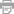# We Are Here Letra

## Alicia Keys

### Otras Canciones

Letra de We Are Here
We are here
We are here for all of us
We are here for all of us
It's why we are here, why we are here
We are here

Tryna get something we never heard
So real but it's so sad
And while we're burning this incense
We gon' pray for the innocent
Cause right now it don't make sense
Right now it don't make sense

Right now it is real
Let's talk Nigeria, and the mass hysteria
Our souls are brought together so that we could love each other brother

We are here
We are here for all of us
We are here for all of us
It's why we are here, why we are here
We are here

But yet Crime is a problem
He wanna shine, he wanna rob 'em
Single mother, where they come from?
How we gonna save the nation
With no support for education
Cause right now it don't make sense
Right now it don't make sense

Let's do more giving
Do more forgiving, yeah
Our souls are brought together so that we can love each other sister

We are here
We are here for all of us
We are here for all of us
It's why we are here, why we are here
We are here

We are here
We are here for all of us
We are here for all of us
It's why we are here, why we are here
We are here

Oh oh oh oh
Oh oh oh oh

We are here
We are here for all of us
We are here for all of us
It's why we are here, why we are here
We are here

We are here
We are here for all of us
We are here for all of us
It's why we are here, why we are here
We are here
•Imprimir Letra